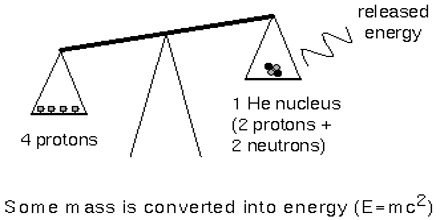Physics

# How to Converting Mass to Energy?General purpose of this lecture is to present on How to Converting Mass to Energy. Out of the special theory of relativity comes the most famous equation in science: E=mc². This equation tells us that mass (m) is just another form of energy (E). The c² is the square of the speed of light. 1 gram of matter is equivalent to the energy obtained by burning 15,000 barrels of oil. We rearrange the protons and neutrons in nuclei to get a lower-mass configuration. The difference between initial mass and final mass is converted to energy.